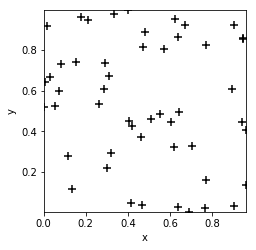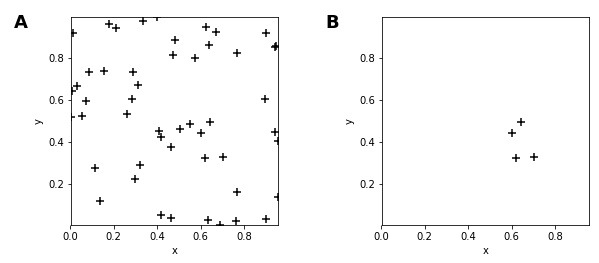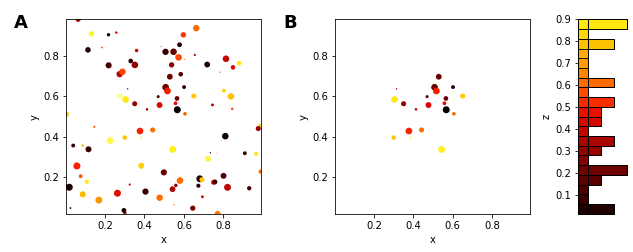# Points¶

Title
Points Element
Dependencies
Matplotlib
Backends
Matplotlib
Bokeh
In :
import numpy as np
import holoviews as hv
from holoviews import dim, opts

hv.extension('matplotlib')The Points element visualizes as markers placed in a space of two independent variables, traditionally denoted x and y. In HoloViews, the names 'x' and 'y' are used as the default key dimensions (kdims) of the element. We can see this from the default axis labels when visualizing a simple Points element:

In :
np.random.seed(12)
coords = np.random.rand(50,2)
points = hv.Points(coords)

points.opts(color='k', marker='+', s=50)

Out:Here both the random x values and random y values are both considered to be the coordinates with no dependency between them (compare this to how Scatter elements are defined). You can think of Points as simply marking positions in some two-dimensional space that can be sliced by specifying a 2D region-of-interest:

In :
points + points[0.6:0.8,0.2:0.5]

Out:Although the simplest Points element simply mark positions in a two-dimensional space without any associated value this doesn't mean value dimensions aren't supported. Here is an example with two additional quantities for each point, declared as the vdimss z and size visualized as the color and size of the dots, respectively:

In :
np.random.seed(10)
data = np.random.rand(100,4)

points = hv.Points(data, vdims=['z', 'size'])
(points + points[0.3:0.7, 0.3:0.7].hist()).opts(
opts.Points(color='z', s=dim('size')*50))

Out:In the right subplot, the hist method is used to show the distribution of samples along the first value dimension we added (z).

The marker shape specified above can be any supported by matplotlib, e.g. s, d, or o; the other options select the color and size of the marker. For convenience with the bokeh backend, the matplotlib marker options are supported using a compatibility function in HoloViews.

Note: Although the Scatter element is superficially similar to the Points element (they can generate plots that look identical), the two element types are semantically quite different. The fundamental difference is that Points are used to visualize data where the y variable is dependent. This semantic difference also explains why the histogram generated by hist call above visualizes the distribution of a different dimension than it does for Scatter.

This difference means that Points naturally combine elements that express independent variables in two-dimensional space, for instance Raster types such as Image. Similarly, Scatter expresses a dependent relationship in two-dimensions and combine naturally with Chart types such as Curve.

For full documentation and the available style and plot options, use hv.help(hv.Points).